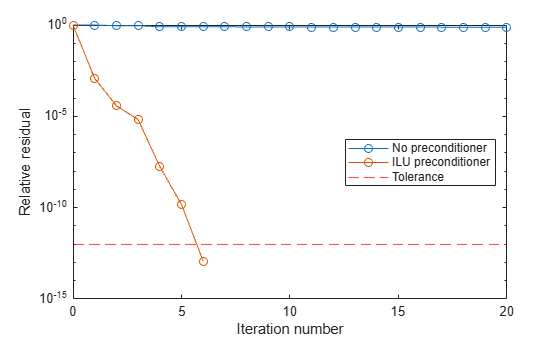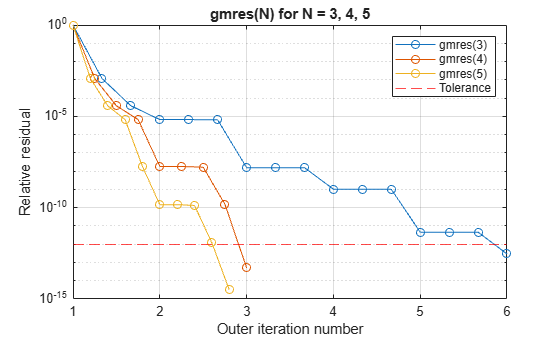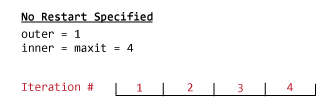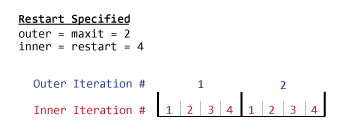# gmres

Solve system of linear equations — generalized minimum residual method

## Syntax

``x = gmres(A,b)``
``x = gmres(A,b,restart)``
``x = gmres(A,b,restart,tol)``
``x = gmres(A,b,restart,tol,maxit)``
``x = gmres(A,b,restart,tol,maxit,M)``
``x = gmres(A,b,restart,tol,maxit,M1,M2)``
``x = gmres(A,b,restart,tol,maxit,M1,M2,x0)``
``[x,flag] = gmres(___)``
``[x,flag,relres] = gmres(___)``
``[x,flag,relres,iter] = gmres(___)``
``[x,flag,relres,iter,resvec] = gmres(___)``

## Description

example

````x = gmres(A,b)` attempts to solve the system of linear equations `A*x = b` for `x` using the Generalized Minimum Residual Method. When the attempt is successful, `gmres` displays a message to confirm convergence. If `gmres` fails to converge after the maximum number of iterations or halts for any reason, it displays a diagnostic message that includes the relative residual `norm(b-A*x)/norm(b)` and the iteration number at which the method stopped. For this syntax, `gmres` does not restart; the maximum number of iterations is `min(size(A,1),10)`.```

example

````x = gmres(A,b,restart)` restarts the method every `restart` inner iterations. The maximum number of outer iterations is ```outer = min(size(A,1)/restart,10)```. The maximum number of total iterations is `restart*outer`, since `gmres` performs `restart` inner iterations for each outer iteration. If `restart` is `size(A,1)` or `[]`, then `gmres` does not restart and the maximum number of total iterations is `min(size(A,1),10)`.```

example

````x = gmres(A,b,restart,tol)` specifies a tolerance for the method. The default tolerance is `1e-6`.```

example

````x = gmres(A,b,restart,tol,maxit)` specifies the maximum number of outer iterations such that the total number of iterations does not exceed `restart*maxit`. If `maxit` is `[]` then `gmres` uses the default, `min(size(A,1)/restart,10)`. If `restart` is `size(A,1)` or `[]`, then the maximum number of total iterations is `maxit` (instead of `restart*maxit`). `gmres` displays a diagnostic message if it fails to converge within the maximum number of total iterations.```

example

````x = gmres(A,b,restart,tol,maxit,M)` specifies a preconditioner matrix `M` and computes `x` by effectively solving the system ${M}^{-1}Ax={M}^{-1}b$. Using a preconditioner matrix can improve the numerical properties of the problem and the efficiency of the calculation.```

example

````x = gmres(A,b,restart,tol,maxit,M1,M2)` specifies factors of the preconditioner matrix `M` such that ```M = M1*M2```.```

example

````x = gmres(A,b,restart,tol,maxit,M1,M2,x0)` specifies an initial guess for the solution vector `x`. The default is a vector of zeros.```

example

````[x,flag] = gmres(___)` returns a flag that specifies whether the algorithm successfully converged. When `flag = 0`, convergence was successful. You can use this output syntax with any of the previous input argument combinations. When you specify the `flag` output, `gmres` does not display any diagnostic messages.```

example

````[x,flag,relres] = gmres(___)` also returns the relative residual `norm(M\(b-A*x))/norm(M\b)`, which includes the preconditioner matrix `M`. If `flag` is `0`, then `relres <= tol`.```

example

````[x,flag,relres,iter] = gmres(___)` also returns the inner and outer iteration numbers at which `x` was computed as a vector `[outer inner]`. The outer iteration number lies in the range `0 <= iter(1) <= maxit` and the inner iteration number is in the range `0 <= iter(2) <= restart`.```

example

````[x,flag,relres,iter,resvec] = gmres(___)` also returns a vector of the residual norms at each inner iteration, including the first residual `norm(M\(b-A*x0))`. These are the residual norms for the preconditioned system.```

## Examples

collapse all

Solve a square linear system using `gmres` with default settings, and then adjust the tolerance and number of iterations used in the solution process.

Create a random sparse matrix `A` with 50% density and nonzeros on the main diagonal. Also create a random vector `b` for the right-hand side of $\mathrm{Ax}=\mathit{b}$.

```rng default A = sprandn(400,400,0.5) + 12*speye(400); b = rand(400,1);```

Solve $\mathrm{Ax}=\mathit{b}$ using `gmres`. The output display includes the value of the relative residual error $\frac{‖\mathit{b}-\mathrm{Ax}‖}{‖\mathit{b}‖}$.

`x = gmres(A,b);`
```gmres stopped at iteration 10 without converging to the desired tolerance 1e-06 because the maximum number of iterations was reached. The iterate returned (number 10) has relative residual 0.35. ```

By default `gmres` uses 10 iterations and a tolerance of `1e-6`, and the algorithm is unable to converge in those 10 iterations for this matrix. Since the residual is still large, it is a good indicator that more iterations (or a preconditioner matrix) are needed. You also can use a larger tolerance to make it easier for the algorithm to converge.

Solve the system again using a tolerance of `1e-4` and 100 iterations.

```tol = 1e-4; maxit = 100; x = gmres(A,b,[],tol,maxit);```
```gmres stopped at iteration 100 without converging to the desired tolerance 0.0001 because the maximum number of iterations was reached. The iterate returned (number 100) has relative residual 0.0045. ```

Even with a looser tolerance and more iterations the residual error does not improve enough for convergence. When an iterative algorithm stalls in this manner it is a good indication that a preconditioner matrix is needed. However, `gmres` also has an input that controls the number of inner iterations. By specifying a value for the inner iterations, `gmres` does more work per outer iteration.

Solve the system again using a `restart` value of 100 and a `maxit` value of 20. Rather than doing 100 iterations once, `gmres` performs 100 iterations between restarts and repeats this 20 times.

```restart = 100; maxit = 20; x = gmres(A,b,restart,tol,maxit);```
```gmres(100) converged at outer iteration 2 (inner iteration 75) to a solution with relative residual 9.3e-05. ```

In this case specifying a large restart value for `gmres` enables it to converge to a solution within the allowed number of iterations. However, large restart values can consume a lot of memory when `A` is also large.

Examine the effect of using a preconditioner matrix with non-restarted `gmres` to solve a linear system.

Load west0479, a real 479-by-479 nonsymmetric sparse matrix.

```load west0479 A = west0479;```

Define `b` so that the true solution to $\mathrm{Ax}=\mathit{b}$ is a vector of all ones.

`b = sum(A,2);`

Set the tolerance and maximum number of iterations.

```tol = 1e-12; maxit = 20;```

Use `gmres` to find a solution at the requested tolerance and number of iterations. Specify five outputs to return information about the solution process:

• `x` is the computed solution to `A*x = b`.

• `fl0` is a flag indicating whether the algorithm converged.

• `rr0` is the relative residual of the computed answer `x`.

• `it0` is a two-element vector `[outer inner]` indicating the inner and outer iteration numbers when `x` was computed.

• `rv0` is a vector of the residual history for $‖\mathit{b}-\mathrm{Ax}‖$.

```[x,fl0,rr0,it0,rv0] = gmres(A,b,[],tol,maxit); fl0```
```fl0 = 1 ```
`rr0`
```rr0 = 0.7603 ```
`it0`
```it0 = 1×2 1 20 ```

`fl0` is 1 because `gmres` does not converge to the requested tolerance `1e-12` within the requested 20 iterations. The best approximate solution that `gmres` returns is the last one (as indicated by `it0(2) = 20`). MATLAB stores the residual history in `rv0`.

To aid with the slow convergence, you can specify a preconditioner matrix. Since `A` is nonsymmetric, use `ilu` to generate the preconditioner $\mathit{M}=\mathit{L}\text{\hspace{0.17em}}\mathit{U}$. Specify a drop tolerance to ignore nondiagonal entries with values smaller than `1e-6`. Solve the preconditioned system ${\mathit{M}}^{-1}\mathit{A}\text{\hspace{0.17em}}\mathit{x}={\mathit{M}}^{-1}\mathit{b}$ by specifying `L` and `U` as inputs to `gmres`. Note that when you specify a preconditioner, `gmres` calculates the residual norm of the preconditioned system for the outputs `rr1` and `rv1`.

```[L,U] = ilu(A,struct('type','ilutp','droptol',1e-6)); [x1,fl1,rr1,it1,rv1] = gmres(A,b,[],tol,maxit,L,U); fl1```
```fl1 = 0 ```
`rr1`
```rr1 = 6.9008e-14 ```
`it1`
```it1 = 1×2 1 6 ```

The use of an `ilu` preconditioner produces a relative residual less than the prescribed tolerance of `1e-12` at the sixth iteration. The first residual `rv1(1)` is `norm(U\(L\b))`, where `M = L*U`. The last residual `rv1(end)` is `norm(U\(L\(b-A*x1)))`.

You can follow the progress of `gmres` by plotting the relative residuals at each iteration. Plot the residual history of each solution with a line for the specified tolerance.

```semilogy(0:length(rv0)-1,rv0/norm(b),'-o') hold on semilogy(0:length(rv1)-1,rv1/norm(U\(L\b)),'-o') yline(tol,'r--'); legend('No preconditioner','ILU preconditioner','Tolerance','Location','East') xlabel('Iteration number') ylabel('Relative residual')```Using a preconditioner with restarted `gmres`.

Load west0479, a real 479-by-479 nonsymmetric sparse matrix.

```load west0479 A = west0479;```

Define `b` so that the true solution to $\mathrm{Ax}=\mathit{b}$ is a vector of all ones.

`b = sum(A,2);`

Construct an incomplete LU preconditioner with a drop tolerance of `1e-6`.

`[L,U] = ilu(A,struct('type','ilutp','droptol',1e-6));`

The benefit to using restarted `gmres` is to limit the amount of memory required to execute the method. Without restart, `gmres` requires `maxit` vectors of storage to keep the basis of the Krylov subspace. Also, `gmres` must orthogonalize against all of the previous vectors at each step. Restarting limits the amount of workspace used and the amount of work done per outer iteration.

Execute `gmres(3)`, `gmres(4)`, and `gmres(5)` using the incomplete LU factors as preconditioners. Use a tolerance of `1e-12` and a maximum of 20 outer iterations.

```tol = 1e-12; maxit = 20; [x3,fl3,rr3,it3,rv3] = gmres(A,b,3,tol,maxit,L,U); [x4,fl4,rr4,it4,rv4] = gmres(A,b,4,tol,maxit,L,U); [x5,fl5,rr5,it5,rv5] = gmres(A,b,5,tol,maxit,L,U); fl3```
```fl3 = 0 ```
`fl4`
```fl4 = 0 ```
`fl5`
```fl5 = 0 ```

`fl3`, `fl4`, and `fl5` are all 0 because in each case restarted `gmres` drives the relative residual to less than the prescribed tolerance of `1e-12`.

The following plot shows the convergence history of each restarted `gmres` method. `gmres(3)` converges at outer iteration 5, inner iteration 3 (`it3 = [5, 3]`) which would be the same as outer iteration 6, inner iteration 0, hence the marking of 6 on the final tick mark.

```semilogy(1:1/3:6,rv3/norm(U\(L\b)),'-o'); h1 = gca; h1.XTick = (1:6); title('gmres(N) for N = 3, 4, 5') xlabel('Outer iteration number'); ylabel('Relative residual'); hold on semilogy(1:1/4:3,rv4/norm(U\(L\b)),'-o'); semilogy(1:1/5:2.8,rv5/norm(U\(L\b)),'-o'); yline(tol,'r--'); hold off legend('gmres(3)','gmres(4)','gmres(5)','Tolerance') grid on```In general the larger the number of inner iterations, the more work `gmres` does per outer iteration and the faster it can converge.

Examine the effect of supplying `gmres` with an initial guess of the solution.

Create a tridiagonal sparse matrix. Use the sum of each row as the vector for the right-hand side of $\mathrm{Ax}=\mathit{b}$ so that the expected solution for $\mathit{x}$ is a vector of ones.

```n = 900; e = ones(n,1); A = spdiags([e 2*e e],-1:1,n,n); b = sum(A,2);```

Use `gmres` to solve $\mathrm{Ax}=\mathit{b}$ twice: one time with the default initial guess, and one time with a good initial guess of the solution. Use 200 iterations and the default tolerance for both solutions. Specify the initial guess in the second solution as a vector with all elements equal to `0.99`.

```maxit = 200; x1 = gmres(A,b,[],[],maxit);```
```gmres converged at iteration 27 to a solution with relative residual 9.5e-07. ```
```x0 = 0.99*e; x2 = gmres(A,b,[],[],maxit,[],[],x0);```
```gmres converged at iteration 7 to a solution with relative residual 6.7e-07. ```

In this case supplying an initial guess enables `gmres` to converge more quickly.

Returning Intermediate Results

You also can use the initial guess to get intermediate results by calling `gmres` in a for-loop. Each call to the solver performs a few iterations and stores the calculated solution. Then you use that solution as the initial vector for the next batch of iterations.

For example, this code performs 100 iterations four times and stores the solution vector after each pass in the for-loop:

```x0 = zeros(size(A,2),1); tol = 1e-8; maxit = 100; for k = 1:4 [x,flag,relres] = gmres(A,b,[],tol,maxit,[],[],x0); X(:,k) = x; R(k) = relres; x0 = x; end```

`X(:,k)` is the solution vector computed at iteration `k` of the for-loop, and `R(k)` is the relative residual of that solution.

Solve a linear system by providing `gmres` with a function handle that computes `A*x` in place of the coefficient matrix `A`.

One of the Wilkinson test matrices generated by `gallery` is a 21-by-21 tridiagonal matrix. Preview the matrix.

`A = gallery('wilk',21)`
```A = 21×21 10 1 0 0 0 0 0 0 0 0 0 0 0 0 0 0 0 0 0 0 0 1 9 1 0 0 0 0 0 0 0 0 0 0 0 0 0 0 0 0 0 0 0 1 8 1 0 0 0 0 0 0 0 0 0 0 0 0 0 0 0 0 0 0 0 1 7 1 0 0 0 0 0 0 0 0 0 0 0 0 0 0 0 0 0 0 0 1 6 1 0 0 0 0 0 0 0 0 0 0 0 0 0 0 0 0 0 0 0 1 5 1 0 0 0 0 0 0 0 0 0 0 0 0 0 0 0 0 0 0 0 1 4 1 0 0 0 0 0 0 0 0 0 0 0 0 0 0 0 0 0 0 0 1 3 1 0 0 0 0 0 0 0 0 0 0 0 0 0 0 0 0 0 0 0 1 2 1 0 0 0 0 0 0 0 0 0 0 0 0 0 0 0 0 0 0 0 1 1 1 0 0 0 0 0 0 0 0 0 0 ⋮ ```

The Wilkinson matrix has a special structure, so you can represent the operation `A*x` with a function handle. When `A` multiplies a vector, most of the elements in the resulting vector are zeros. The nonzero elements in the result correspond with the nonzero tridiagonal elements of `A`. Moreover, only the main diagonal has nonzeros that are not equal to 1.

The expression $\mathrm{Ax}$ becomes:

$\mathrm{Ax}=\left[\begin{array}{cccccccccc}10& 1& 0& \cdots & & \cdots & & \cdots & 0& 0\\ 1& 9& 1& 0& & & & & & 0\\ 0& 1& 8& 1& 0& & & & & ⋮\\ ⋮& 0& 1& 7& 1& 0& & & & \\ & & 0& 1& 6& 1& 0& & & ⋮\\ ⋮& & & 0& 1& 5& 1& 0& & \\ & & & & 0& 1& 4& 1& 0& ⋮\\ ⋮& & & & & 0& 1& 3& \ddots & 0\\ 0& & & & & & 0& \ddots & \ddots & 1\\ 0& 0& \cdots & & \cdots & & \cdots & 0& 1& 10\end{array}\right]\left[\begin{array}{c}{\mathit{x}}_{1}\\ {\mathit{x}}_{2}\\ {\mathit{x}}_{3}\\ {\mathit{x}}_{4}\\ {\mathit{x}}_{5}\\ ⋮\\ \\ ⋮\\ \\ {\mathit{x}}_{21}\end{array}\right]=\left[\begin{array}{c}10{\mathit{x}}_{1}+{\mathit{x}}_{2}\\ {\mathit{x}}_{1}+9{\mathit{x}}_{2}+{\mathit{x}}_{3}\\ {\mathit{x}}_{2}+8{\mathit{x}}_{3}+{\mathit{x}}_{4}\\ ⋮\\ {\mathit{x}}_{19}+9{\mathit{x}}_{20}+{\mathit{x}}_{21}\\ {\mathit{x}}_{20}+10{\mathit{x}}_{21}\end{array}\right]$.

The resulting vector can be written as the sum of three vectors:

$\mathrm{Ax}=\left[\begin{array}{c}0+10{\mathit{x}}_{1}+{\mathit{x}}_{2}\\ {\mathit{x}}_{1}+9{\mathit{x}}_{2}+{\mathit{x}}_{3}\\ {\mathit{x}}_{2}+8{\mathit{x}}_{3}+{\mathit{x}}_{4}\\ ⋮\\ {\mathit{x}}_{19}+9{\mathit{x}}_{20}+{\mathit{x}}_{21}\\ {\mathit{x}}_{20}+10{\mathit{x}}_{21}+0\end{array}\right]$=$\left[\begin{array}{c}0\\ {\mathit{x}}_{1}\\ ⋮\\ {\mathit{x}}_{20}\end{array}\right]+\left[\begin{array}{c}10{\mathit{x}}_{1}\\ 9{\mathit{x}}_{2}\\ ⋮\\ 10{\mathit{x}}_{21}\end{array}\right]+\left[\begin{array}{c}{\mathit{x}}_{2}\\ ⋮\\ {\mathit{x}}_{21}\\ 0\end{array}\right]$.

In MATLAB®, write a function that creates these vectors and adds them together, thus giving the value of `A*x`:

```function y = afun(x) y = [0; x(1:20)] + ... [(10:-1:0)'; (1:10)'].*x + ... [x(2:21); 0]; end ```

(This function is saved as a local function at the end of the example.)

Now, solve the linear system $\mathrm{Ax}=\mathit{b}$ by providing `gmres` with the function handle that calculates `A*x`. Use a tolerance of `1e-12`, 15 outer iterations, and 10 inner iterations before restart.

```b = ones(21,1); tol = 1e-12; maxit = 15; restart = 10; x1 = gmres(@afun,b,restart,tol,maxit)```
```gmres(10) converged at outer iteration 5 (inner iteration 10) to a solution with relative residual 5.3e-13. ```
```x1 = 21×1 0.0910 0.0899 0.0999 0.1109 0.1241 0.1443 0.1544 0.2383 0.1309 0.5000 ⋮ ```

Check that `afun(x1)` produces a vector of ones.

`afun(x1)`
```ans = 21×1 1.0000 1.0000 1.0000 1.0000 1.0000 1.0000 1.0000 1.0000 1.0000 1.0000 ⋮ ```

Local Functions

```function y = afun(x) y = [0; x(1:20)] + ... [(10:-1:0)'; (1:10)'].*x + ... [x(2:21); 0]; end```

## Input Arguments

collapse all

Coefficient matrix, specified as a square matrix or function handle. This matrix is the coefficient matrix in the linear system `A*x = b`. Generally, `A` is a large sparse matrix or a function handle that returns the product of a large sparse matrix and column vector.

#### Specifying `A` as a Function Handle

You can optionally specify the coefficient matrix as a function handle instead of a matrix. The function handle returns matrix-vector products instead of forming the entire coefficient matrix, making the calculation more efficient.

To use a function handle, use the function signature `function y = afun(x)`. Parameterizing Functions explains how to provide additional parameters to the function `afun`, if necessary. The function call `afun(x)` must return the value of `A*x`.

Data Types: `double` | `function_handle`
Complex Number Support: Yes

Right-hand side of linear equation, specified as a column vector. The length of `b` must be equal to `size(A,1)`.

Data Types: `double`
Complex Number Support: Yes

Number of inner iterations before restart, specified as a scalar integer. Use this input along with the `maxit` input to control the maximum number of iterations, `restart*maxit`. If `restart` is `[]` or `size(A,1)`, then `gmres` does not restart and the total number of iterations is `maxit`.

A large `restart` value typically leads to better convergence behavior, but also has higher time and memory requirements.

Data Types: `double`

Method tolerance, specified as a positive scalar. Use this input to trade-off accuracy and runtime in the calculation. `gmres` must meet the tolerance within the number of allowed iterations to be successful. A smaller value of `tol` means the answer must be more precise for the calculation to be successful.

Data Types: `double`

Maximum number of outer iterations, specified as a positive scalar integer. Increase the value of `maxit` to allow more iterations for `gmres` to meet the tolerance `tol`. Generally, the smaller the value of `tol`, the more iterations are required to successfully complete the calculation.

If the `restart` input is also specified, then the total number of iterations is `restart*maxit`. Otherwise, the total number of iterations is `maxit`.

The default value of `maxit` depends on whether `restart` is specified:

• If `restart` is unspecified, or specified as `[]` or `size(A,1)`, then the default value of `maxit` is `min(size(A,1),10)`.

• If `restart` is specified as a value in the range ```1 <= restart < size(A,1)```, then the default value of `maxit` is `min(ceil(size(A,1)/restart),10)`.

Data Types: `double`

Preconditioner matrices, specified as separate arguments of matrices or function handles. You can specify a preconditioner matrix `M` or its matrix factors `M = M1*M2` to improve the numerical aspects of the linear system and make it easier for `gmres` to converge quickly. You can use the incomplete matrix factorization functions `ilu` and `ichol` to generate preconditioner matrices. You also can use `equilibrate` prior to factorization to improve the condition number of the coefficient matrix. For more information on preconditioners, see Iterative Methods for Linear Systems.

`gmres` treats unspecified preconditioners as identity matrices.

#### Specifying `M` as a Function Handle

You can optionally specify any of `M`, `M1`, or `M2` as function handles instead of matrices. The function handle performs matrix-vector operations instead of forming the entire preconditioner matrix, making the calculation more efficient.

To use a function handle, use the function signature ```function y = mfun(x)```. Parameterizing Functions explains how to provide additional parameters to the function `mfun`, if necessary. The function call `mfun(x)` must return the value of `M\x` or `M2\(M1\x)`.

Data Types: `double` | `function_handle`
Complex Number Support: Yes

Initial guess, specified as a column vector with length equal to `size(A,2)`. If you can provide `gmres` with a more reasonable initial guess `x0` than the default vector of zeros, then it can save computation time and help the algorithm converge faster.

Data Types: `double`
Complex Number Support: Yes

## Output Arguments

collapse all

Linear system solution, returned as a column vector. This output gives the approximate solution to the linear system `A*x = b`. If the calculation is successful (`flag = 0`), then `relres` is less than or equal to `tol`.

Whenever the calculation is not successful (`flag ~= 0`), the solution `x` returned by `gmres` is the one with minimal residual norm computed over all the iterations.

Convergence flag, returned as one of the scalar values in this table. The convergence flag indicates whether the calculation was successful and differentiates between several different forms of failure.

Flag Value

Convergence

`0`

Success — `gmres` converged to the desired tolerance `tol` within `maxit` iterations.

`1`

Failure — `gmres` iterated `maxit` iterations but did not converge.

`2`

Failure — The preconditioner matrix `M` or `M = M1*M2` is ill conditioned.

`3`

Failure — `gmres` stagnated after two consecutive iterations were the same.

`4`

Failure — One of the scalar quantities calculated by the `gmres` algorithm became too small or too large to continue computing.

Relative residual error, returned as a scalar. The relative residual error `relres = norm(M\(b-A*x))/norm(M\b)` is an indication of how accurate the answer is. Note that `gmres` includes the preconditioner matrix `M` in the relative residual calculation, while most other iterative solvers do not. If the calculation converges to the tolerance `tol` within `maxit` iterations, then ```relres <= tol```.

Data Types: `double`

Outer and inner iteration numbers, returned as a two-element vector ```[outer inner]```. This output indicates the inner and outer iteration numbers at which the computed answer for `x` was calculated:

• If `restart` is unspecified, or specified as `[]` or `size(A,1)`, then ```outer = 1``` and all iterations are considered to be inner iterations.

• If `restart` is specified as a value in the range ```1 <= restart < size(A,1)```, then the outer iteration number is in the range `0 <= outer <= maxit` and the inner iteration number is in the range `0 <= inner <= restart`.

Data Types: `double`

Residual error, returned as a vector. The residual error `norm(M\(b-A*x))` reveals how close the algorithm is to converging for a given value of `x`. Note that `gmres` includes the preconditioner matrix `M` in the relative residual calculation, while most other iterative solvers do not. The number of elements in `resvec` is equal to the total number of iterations (if `restart` is used, this is at most `restart*maxit`). You can examine the contents of `resvec` to help decide whether to change the values of `restart`, `tol`, or `maxit`.

Data Types: `double`

collapse all

### Generalized Minimum Residual Method

The generalized minimum residual (GMRES) algorithm was developed to extend the minimum residual (MINRES) algorithm to unsymmetric matrices.

Like conjugate gradients (CG) methods, the GMRES algorithm computes orthogonal sequences, but GMRES needs to store all previous vectors in the sequences. This storage of previous vectors can consume a lot of memory if left unchecked. The "restarted" version of the algorithm controls storage of these sequences by periodically clearing the intermediate sequences and using the results as the initial value in another iteration.

Choosing an appropriate "restart" value is essential to good performance, but choosing such a value is mostly a matter of experience. If the number of iterations before restart is too small, the algorithm might be very slow to converge or fail to converge entirely. But if the restart value is too large, then the algorithm has increased storage requirements and might do unnecessary work .

### Inner and Outer Iterations

Inner iterations are the iterations that `gmres` completes before restarting. You can specify the number of inner iterations with the `restart` argument.

Each time `gmres` restarts, the outer iteration number advances. You can specify the maximum number of outer iterations with the `maxit` argument. The default number of outer iterations is `min(size(A,1)/restart,10)`.

For example, if you do not specify `restart`, then the maximum number of iterations is determined by the value of `maxit`, and `gmres` does not restart:However, when you specify `restart`, the `gmres` function performs several inner iterations (specified by `restart`) for each outer iteration (specified by `maxit`). In this case, the maximum number of total iterations is `restart*maxit`:## Tips

• Convergence of most iterative methods depends on the condition number of the coefficient matrix, `cond(A)`. You can use `equilibrate` to improve the condition number of `A`, and on its own this makes it easier for most iterative solvers to converge. However, using `equilibrate` also leads to better quality preconditioner matrices when you subsequently factor the equilibrated matrix ```B = R*P*A*C```.

• You can use matrix reordering functions such as `dissect` and `symrcm` to permute the rows and columns of the coefficient matrix and minimize the number of nonzeros when the coefficient matrix is factored to generate a preconditioner. This can reduce the memory and time required to subsequently solve the preconditioned linear system.

 Barrett, R., M. Berry, T. F. Chan, et al., Templates for the Solution of Linear Systems: Building Blocks for Iterative Methods, SIAM, Philadelphia, 1994.

 Saad, Yousef and Martin H. Schultz, “GMRES: A generalized minimal residual algorithm for solving nonsymmetric linear systems,” SIAM J. Sci. Stat. Comput., July 1986, Vol. 7, No. 3, pp. 856-869.